# 1. Fundamental Interval and Ratio Scales

• For physical attributes, objects are fundamentally measurable, the properties of order and addition have a physical analogue (類比).
• Order. One rod is observed to be longer than another.
• Addition. Two (equal) rods can be added. If their composite length equals a longer rod, then the length of the longer must be twice the length of the shorter rods.

# 2. Conjoint Measurement Theory

• The theory of conjoint measurement (Luce & Tuckey, 1964). .font60[(提出 Tukey’s HSD test 的那位 Tukey!)] specifies conditions that can establish the required properties of order and additivity for interval-scale measurement.
• Conjoint measurement is obtained when an outcome variable is an additive function of two other variables, assuming that all three variables may be ordered for magnitude.

[p.141]

# 3. Rasch’s Example

Conjoint measurement theory may be applied to Rasch’s (1960) favorite example as follows.

\begin{align*} \rm Acceleration &= \rm \dfrac{Force}{Mass} \\ \rm \log (Acceleration) &= \rm \log (Force) - \log (Mass) \\ \end{align*}

For Rasch’s 1PL model, the item performance scaled as log odds is an additive combination of log trait level, $$\theta_s$$, and log item difficulty, $$\beta_i$$.

$\rm \log (Item \ Odds) = \log (Trait \ Level) - \log (Item \ Difficulty) \\$

[Eq. 6.6-6.8]

## wiki The definition of measurement

• In physics and metrology, the standard definition of measurement is the estimation of the ratio between a magnitude of a continuous quantity and a unit magnitude of the same kind (de Boer, 1994/95; Emerson, 2008).
e.g., “The hallway is 4 m long”.
• For some other quantities, Invariant are ratios b/w attribute differences. e.g., the Fahrenheit or Celsius scales.
• What are really being measured with such instruments are the magnitudes of temperature differences.
e.g., the unit of the Celsius scale is 1/100th of the difference in temperature between the freezing and boiling points of water at sea level.

## wiki Extensive and intensive quantity

• Extensive (外延性). Length, or Mass.
e.g., 60(g) 饅頭剝成兩份 -> 30(g)+30(g)
• Intensive (內含性) . Temperature.
e.g., 60(°C) 饅頭剝成兩份 -> 30(°C)+30(°C) ??
(i.e., 溫度不具有可加性)
• Psychological attributes?? like temperature, or like length?? The theory of conjoint measurement provides a theoretical means of dealing w/ this.

[See: Theory of conjoint measurement from Wikipedia]

# 4. Fundamental Measurement and the Rasch Model

Rasch model derived from several conditions for scores. - the sufficiency of the unweighted total score (Fisher, 1995) - consistency of ordering persons and items (Roskam & Jansen, 1984) - additivity (Andrich, 1988) - the principle of specific objectivity (Rasch, 1977). Comparisons b/w objects must be generalizable beyond the specific conditions under which they were observed.

# 5. Invariant-Person Comparisons

[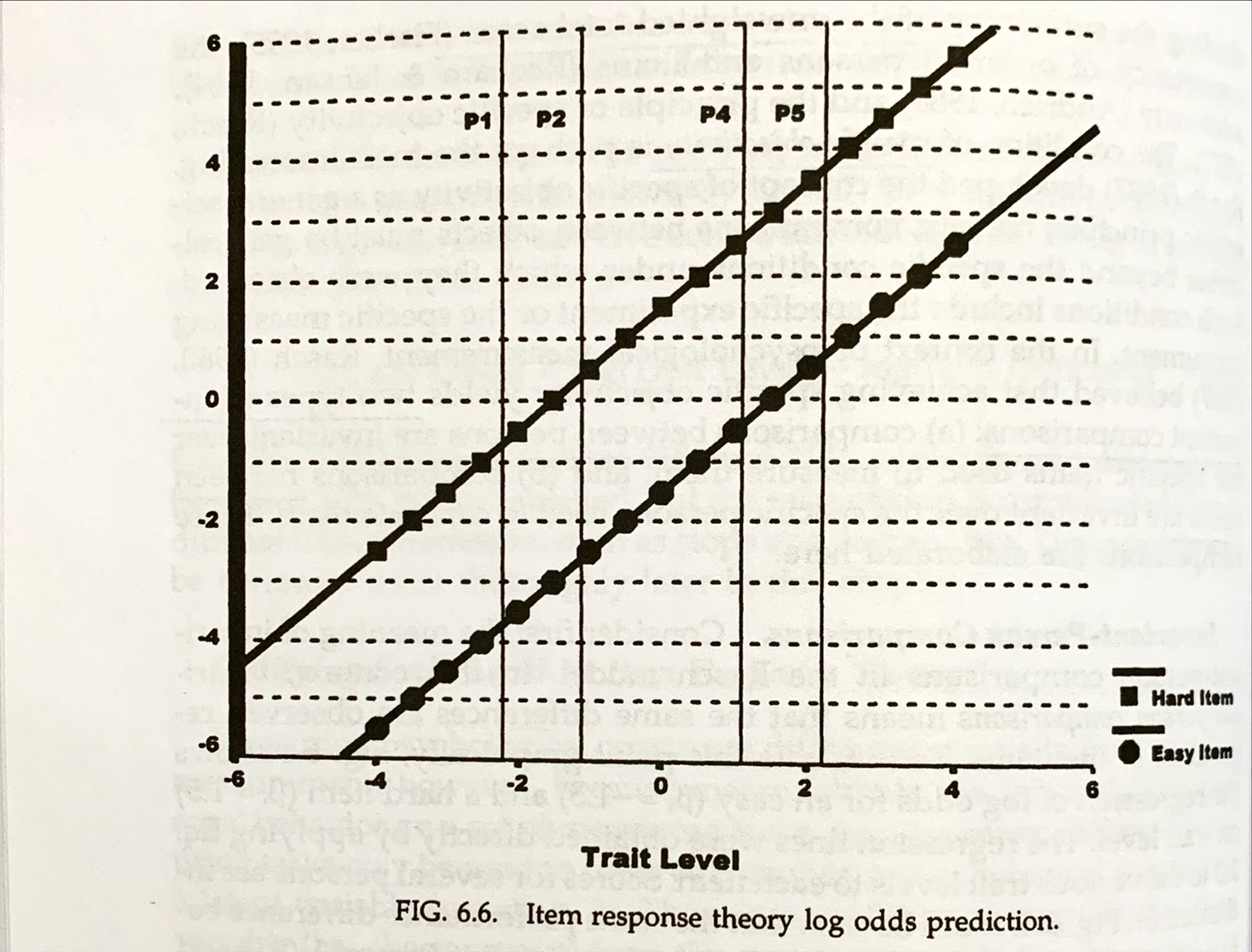] []

## Compare persons at the low/high end.

\begin{align*} \ln \dfrac{P(X_{i1})}{1-P(X_{i1})} - \ln \dfrac{P(X_{i2})}{1-P(X_{i2})} &= (\theta_1 - \beta_i) - ( \theta_2 - \beta_i)\\ &= \theta_1 - \theta_2 \\ &=-2.20-(-1.10) \\ &=-1.10 \end{align*}

\begin{align*} \ln \dfrac{P(X_{i4})}{1-P(X_{i4})} - \ln \dfrac{P(X_{i5})}{1-P(X_{i5})} &= (\theta_4 - \beta_i) - ( \theta_5 - \beta_i)\\ &= \theta_1 - \theta_2 \\ &=1.10-2.20 \\ &=-1.10 \end{align*}

[Eq. 6.10-6.11]

# 6. Invariant-Item Comparisons

• Log odds of item 1 and item 2, for any subjects.

$\ln \dfrac{P(X_{1s})}{1-P(X_{1s})}=\theta_s - \beta_1$ $\ln \dfrac{P(X_{2s})}{1-P(X_{2s})}=\theta_s - \beta_2$

[Eq. 6.12]

## Item comparisons for 1PL/2PL models.

\begin{align*} \ln \dfrac{P(X_{1s})}{1-P(X_{1s})} - \ln \dfrac{P(X_{2s})}{1-P(X_{2s})} &= (\theta_s - \beta_1) - ( \theta_s - \beta_2)\\ &= -(\beta_1 - \beta_2) \end{align*}

For 2PL model, the difference does NOT depend only on item difficulty (i.e. we also need to consider $$\theta_s$$, $$\alpha_1$$, and $$\alpha_2$$).

\begin{align*} &\ln \dfrac{P(X_{1s})}{1-P(X_{1s})} - \ln \dfrac{P(X_{2s})}{1-P(X_{2s})} \\ &= \alpha_1(\theta_s - \beta_1) - \alpha_2( \theta_s - \beta_2)\\ &= \theta_s(\alpha_1 - \alpha_2)-(\alpha_1\beta_1 - \alpha_2\beta_2) \end{align*}

[Eq. 6.13-6.14]

# 7. A Caveat 注意事項

• (O) equating trait levels across non-overlapping item sets, e.g. adaptive testing.
• (O) item parameters estimates are not much influenced by the trait distribution in the calibration sample. (See Whitely & Dawis, 1974).
• (X) the estimates from test data will have identical properties over either items or over persons.
• e.g., if the item set is easy, a low trait level will be more accurately estimated than a high trait level. (information)
Although estimates can be equated over these conditions, the standard errors are influenced. (See Ch. 7, 8, 9)

# 8. Funamental Measurement of Persons in More Complex Models (2PL)

[ \begin{align*} &\ln \dfrac{P(X_{i1})}{1-P(X_{i1})} - \ln \dfrac{P(X_{i2})}{1-P(X_{i2})} \\ &= \alpha_i(\theta_1 - \beta_i) - \alpha_i( \theta_2 - \beta_i)\\ &= \alpha_i(\theta_1 - \theta_2) \\ &= \alpha_i(-2.20-(-1.10)) \\ &=-1.10\alpha_i \end{align*} [Eq. 6.15]]

[]

# 9. Fundamental Measurement and Scale type

Ratio scale –> interval scale.
The odds that a person passes an item is given by the ratio of trait level to item difficulty. Where $$\xi_s = \exp(\theta_s), \epsilon_i=\exp(\beta_i)$$.

$\dfrac{P_{i1}}{1-P_{i1}}=\dfrac{e^{\theta_1}}{e^{\beta_i}}=\dfrac{\xi_1}{\epsilon_i}; \dfrac{P_{i2}}{1-P_{i2}}=\dfrac{\xi_2}{\epsilon_i}$

Consider the Rasch model in the log odds form. $\ln \dfrac{P_{i1}}{1-P_{i1}}=\theta_s-\beta_i$ [Eq. 6.16, 6.18]

The odds ratio of person 1 and person 2 at item i as follows.

$\dfrac{ \dfrac{P_{i1}}{1-P_{i1}} }{ \dfrac{P_{i2}}{1-P_{i2}} }=\dfrac{ \dfrac{\xi_1}{\epsilon_i} }{ \dfrac{\xi_2}{\epsilon_i} } = \dfrac{\xi_1}{\xi_2}$

The relative odds b/w that any item is solved for the 2 persons is simply the ratio of their trait levels.

[Eq. 6.17]

# 10. Evaluating Psychological Data for Fundamental Scalability

Luce and Tukey (1964) outline several conditions that must be obtained to support additivity. (可加性需要幾個條件)

• Solvability and the Archmidean condition. (to ensure continuity) (See also. Theory of conjoint measurement).
• Single cancellation or independence axiom.
• Double cancellation axiom.

Michell (1990) shows how the double canellation condition establishes that two parameters are additivity related to a third variable.

Consider two natural attributes A, and X. It is not known that either A or X is a continuous quantity, or both.

• A: (a, b, c)
• X: (x, y, z)
• P: (a, x), (b, y),…, (c, z)

The quantification of A, X and P depends upon the behaviour of the relation holding upon the levels of P.

[See: Theory of conjoint measurement from Wikipedia]

## wiki Single Cancellation Axiom

The theory of conjoint measurement

It can be seen that a > b because (a, x) > (b, x), (a, y) > (b, y) and (a, z) > (b, z).

[See: Theory of conjoint measurement from Wikipedia]

## wiki Double Cancellation Axiom

Given that: $$(a,y)>(b,x)$$ is true if and only if $$a+y>b+x$$, and $$(b,z)>(c,y)$$ is true if and only if $$b+z>c+y$$, it follows that: $$a+y+b+z>b+x+c+y$$.

Cancelling the common terms results in: $$(a,z)>(c,x)$$.

[See: Theory of conjoint measurement from Wikipedia]

## Double Cancellation Condition (機率值也可以)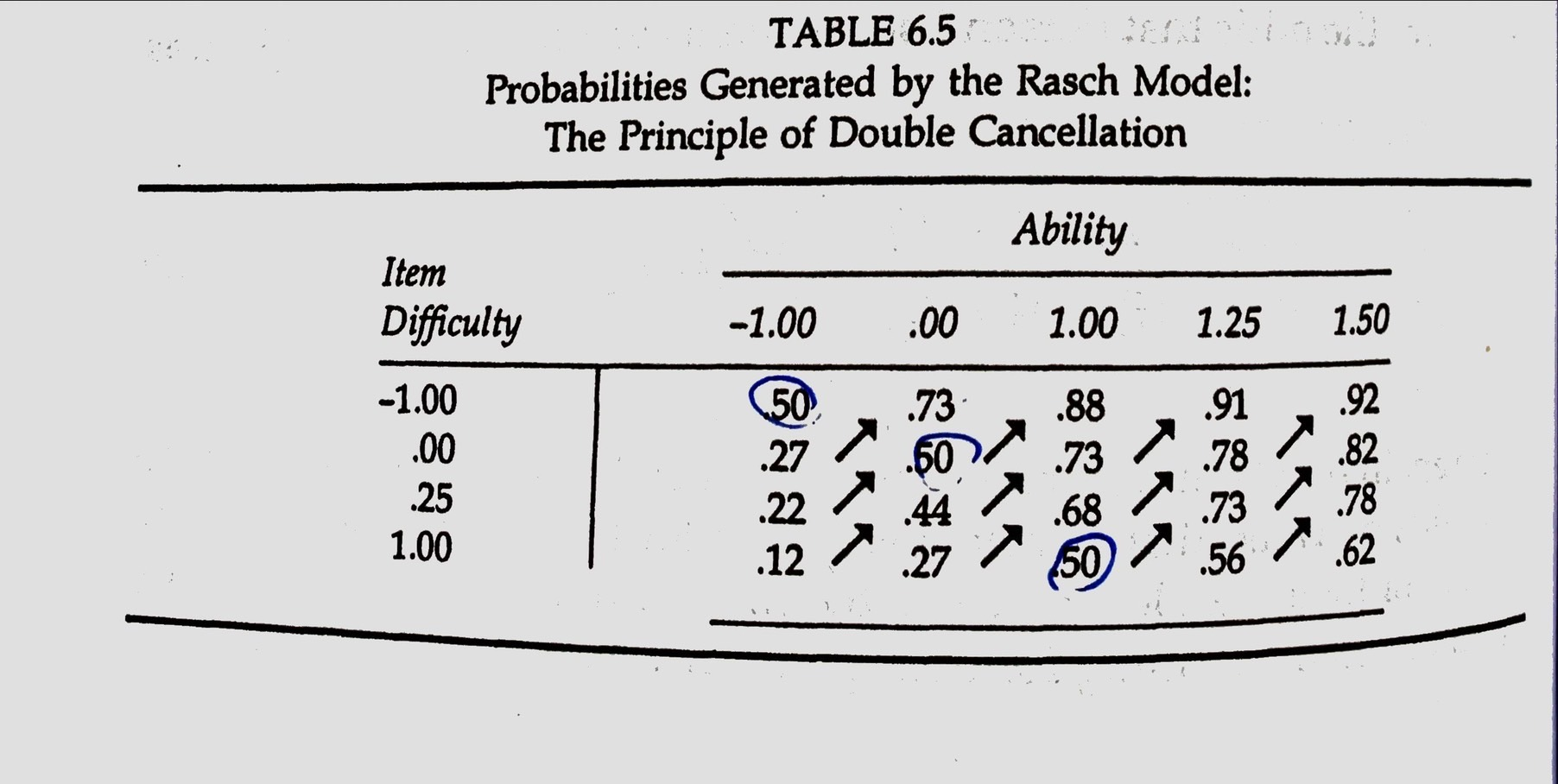.font90[ - Single cancellation. the relative order of probabilities for any two items is the same, regardless of the ability column. Also, the probabilities for persons is the same. - Double cancellation. the third variable (表格中的機率值) increases as both the other two variables (難度、能力) increase.]

[Table 6.5]

with only minor exceptions, these data generally correspond to the double-cancellation pattern.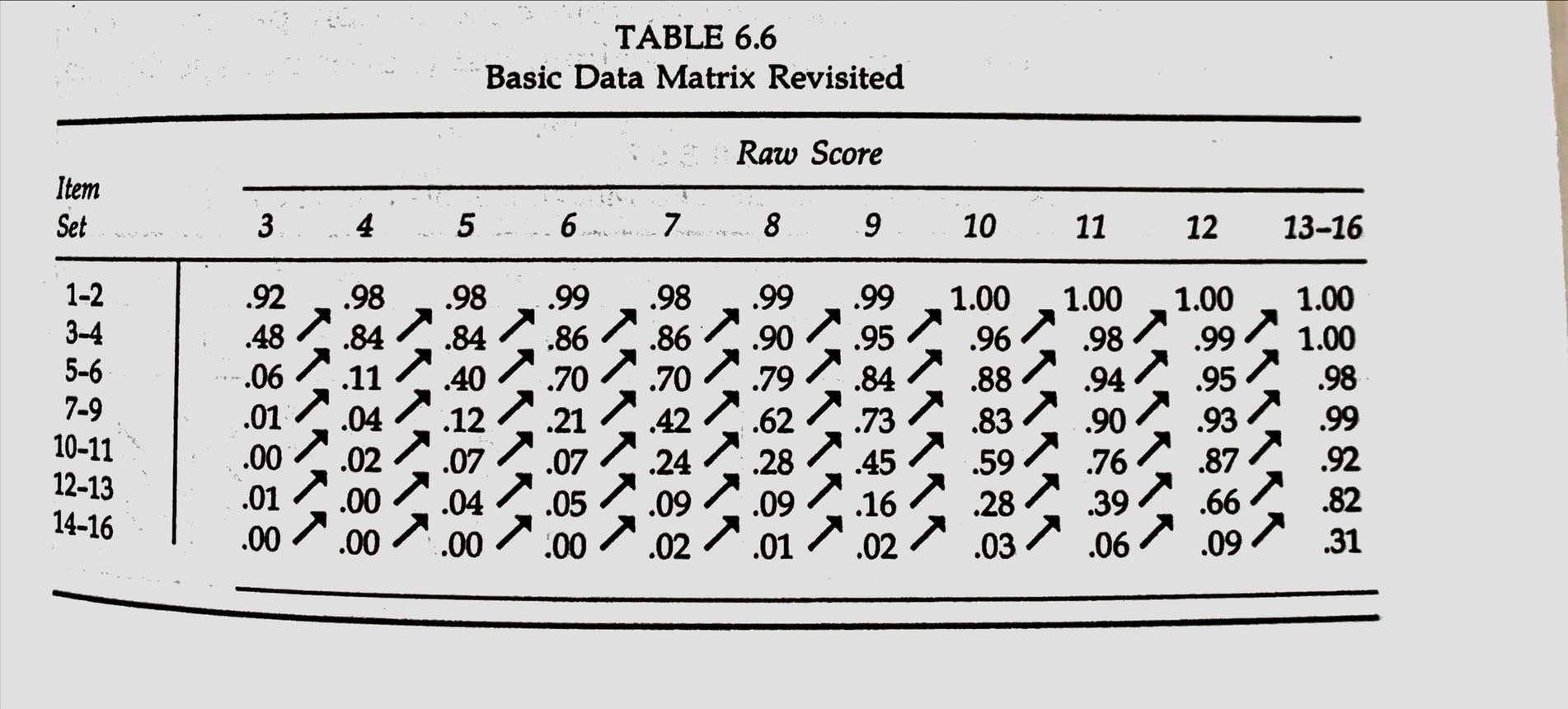[Table 6.6]

## Fig. 3.1 revisited.

[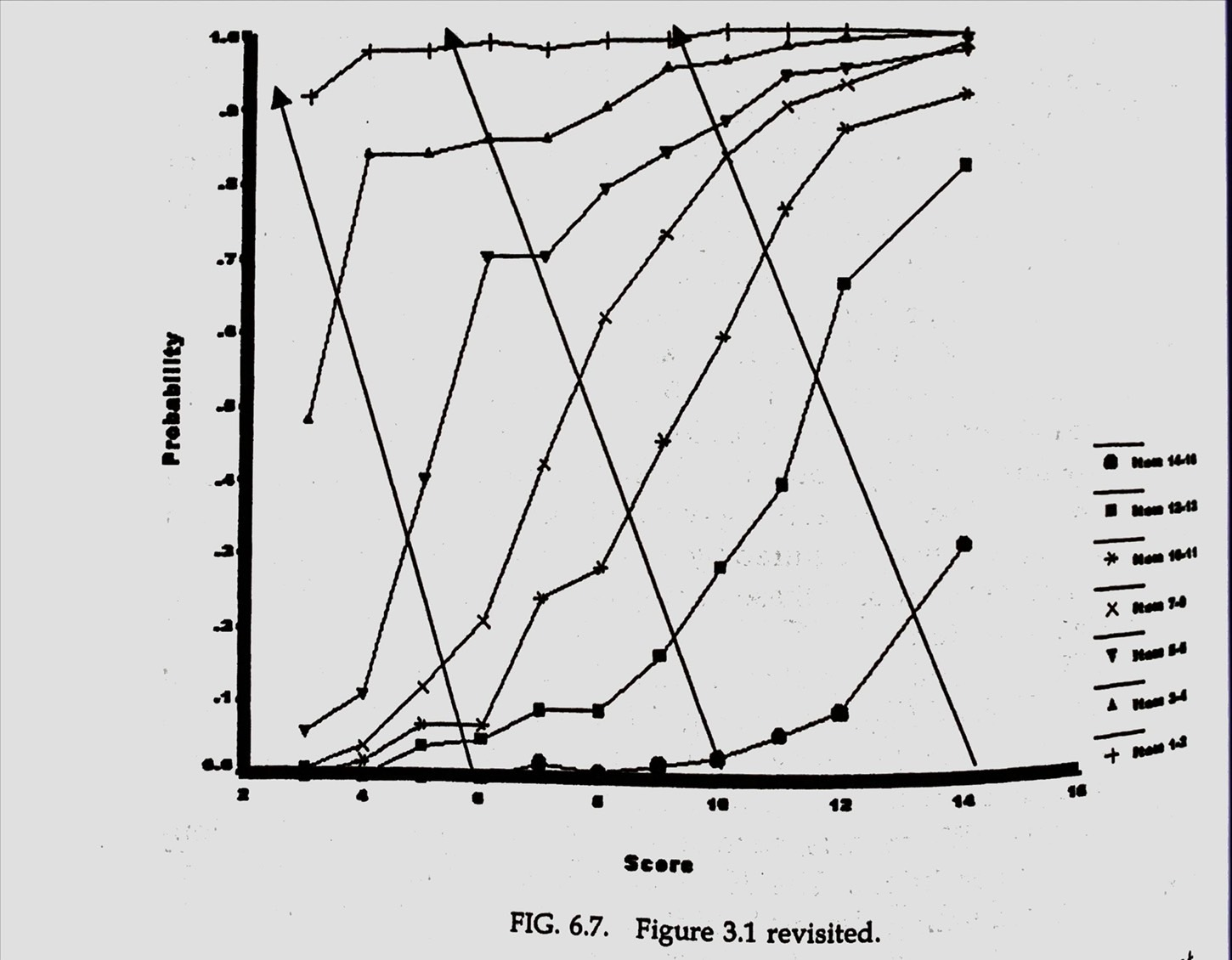[Fig. 6.7]] [ - double cancellation is shown by the diagonal arrows. - double cancellation is equivalent to stating that the ICCs do not cross.]

• More complex IRT model (2PL) do not meet the double-cancellation conditions. (discrimination)
• Conjoint measurement theory is only one view of scale level. The 2PL model may be more favorably evaluated under other views of scale level.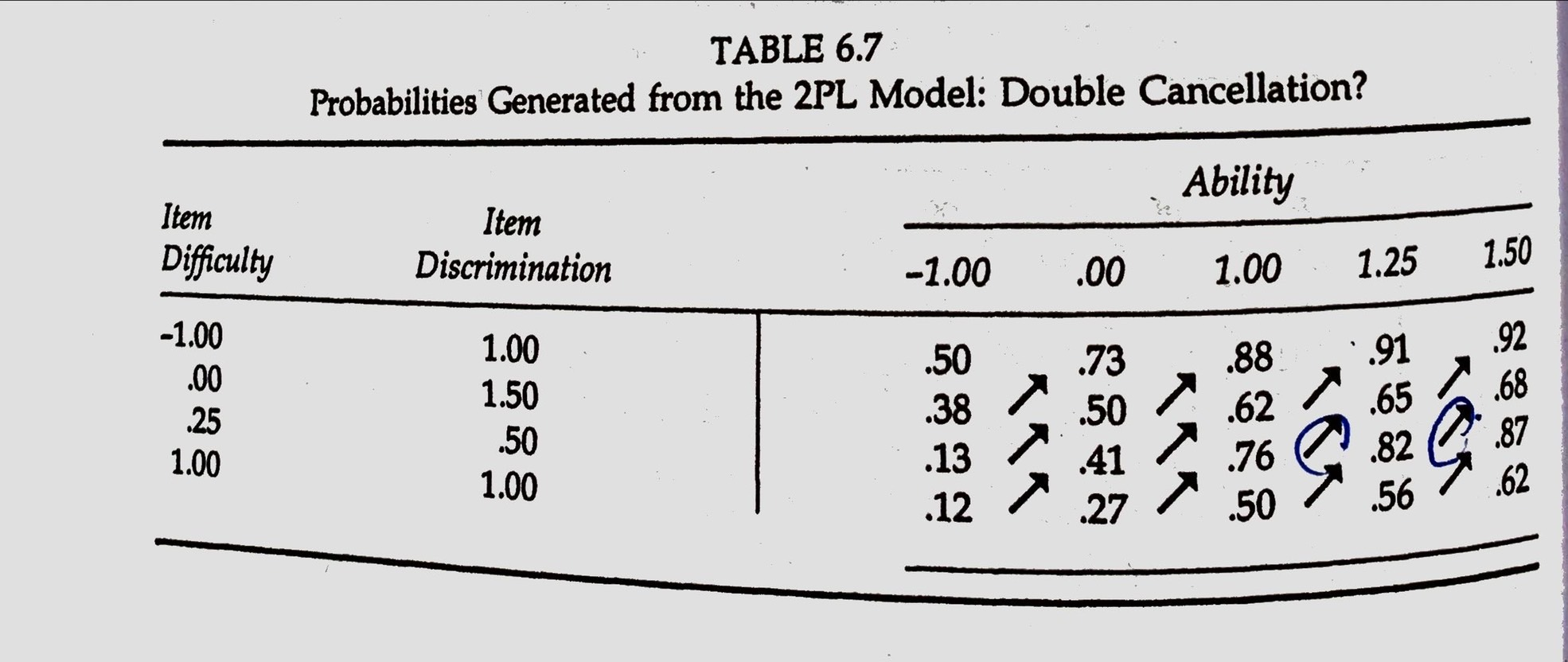[Table 6.7]

# 11. Justifying Scale Level in CTT (1)

The meaning of score differences clearly depends on the test and its item properties. Interval-level can be justified in CTT if two conditions hold, 1. the true trait level , measured on an interval scale, is normally distribution. (assumption) 2. observed scores have a normal distribution.
- items can be selected to yield normal distributions by choosing difficulty levels that are appropriate for the norm group. (.40 ~ .60)
- non-normally distributed observed scores can be normalized.

# 11. Justifying Scale Level in CTT (2)

[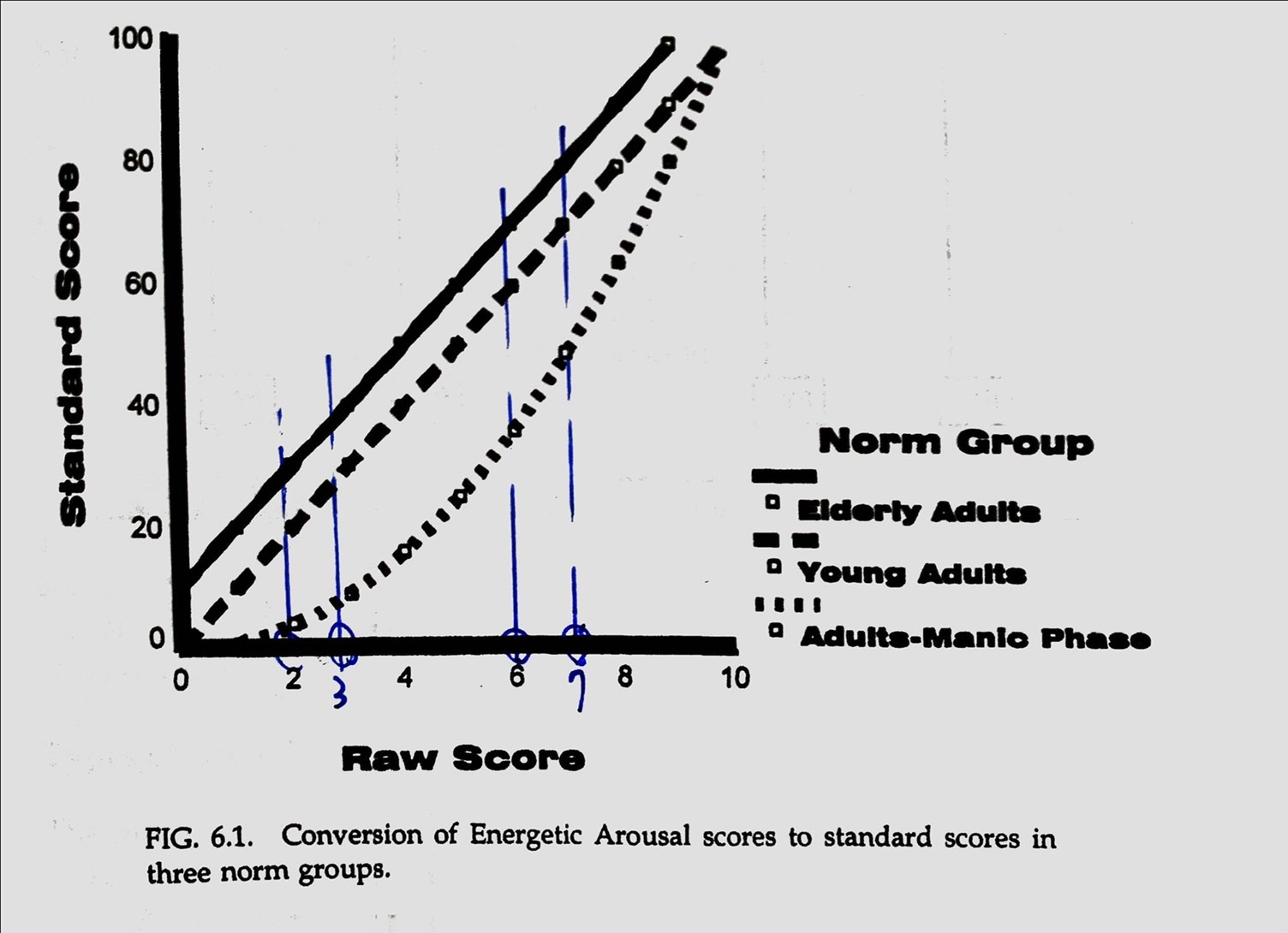]

[ When multiple norm groups exist, it is difficult to justify scaling level on the basis of achieving a certain distribution of scores. ]

# 12. Practical Importance of Scale Level

same data can lead different conclusions. CTT/IRT

• Two groups w/ equal true means can differ significantly on observed means if the observed scores are not linearly related to true score. (Maxwell & Delaney, 1985)
• Significant interactions can be observed from raw scores in factorial ANOVA designs (Embretson, 1997).
• Estimates of growth and learning curves, repeated measures comparisons and even regression coefficients have been shown to depend on the scale level reached for observed scores.

## Mapping proportion correct to trait level

[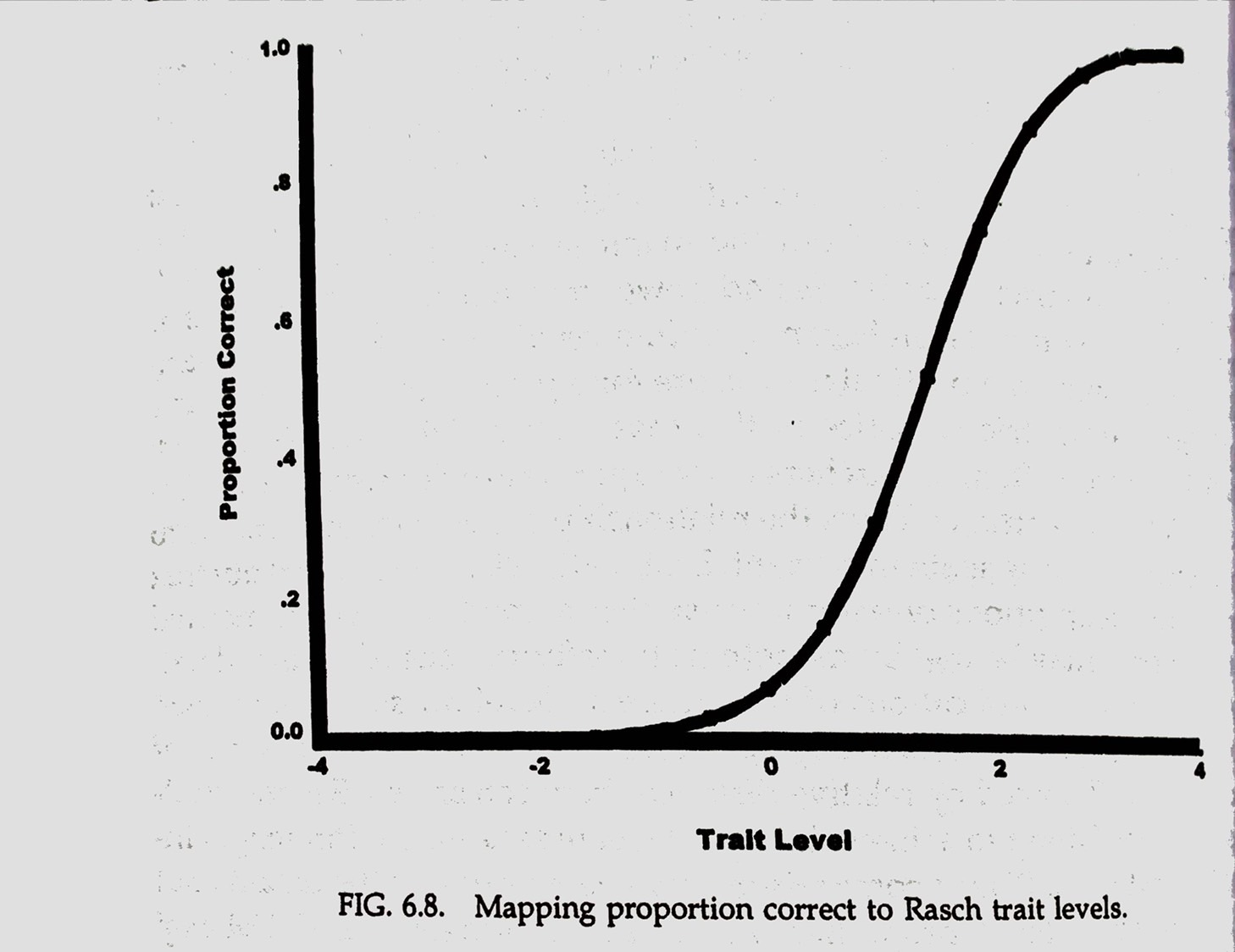]

[]

## A simulation study of 3x2 factorial ANOVA design

• 300 cases per group
• randomly sampled from a distribution w/ variance =1.0
• means of control group. -1 (low), 0 (moderate), 1 (high).
• means of treatment group. -0.5 (low), 0.5 (moderate), 1.5 (high).

Conclusion. The greatest differences b/w conditions will be found for the population for which the level of test difficulty is most appropriate. (high trait w/ hard item, 1.5)

## Means of control and treatment groups for three populations.

[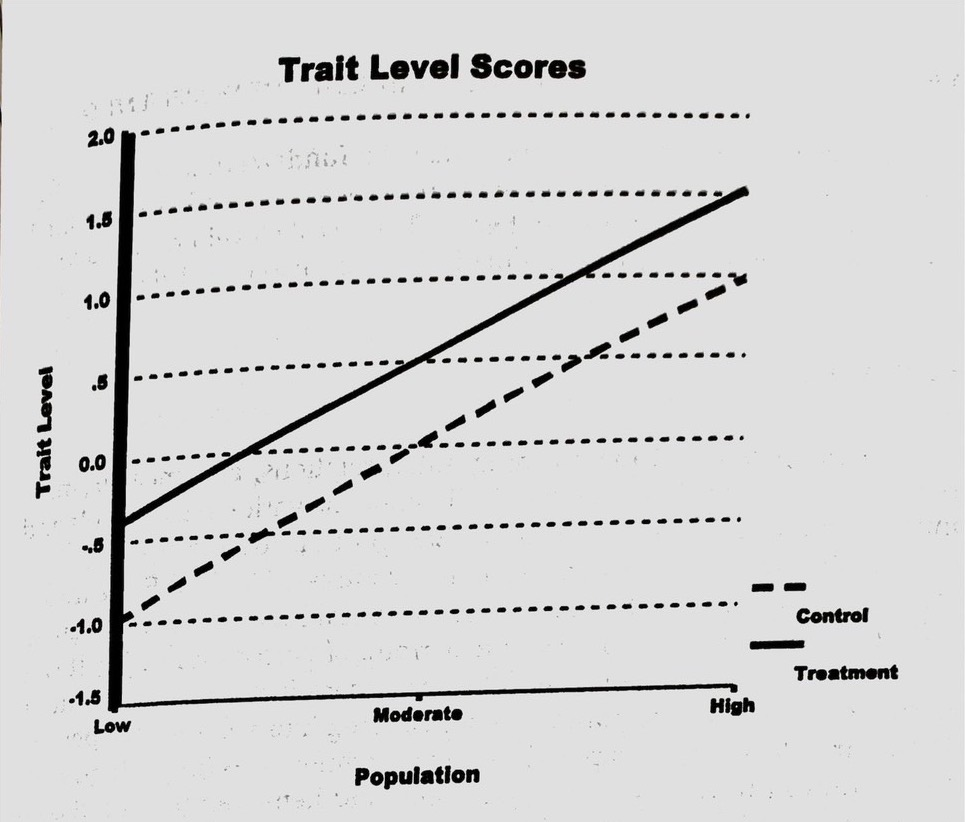] [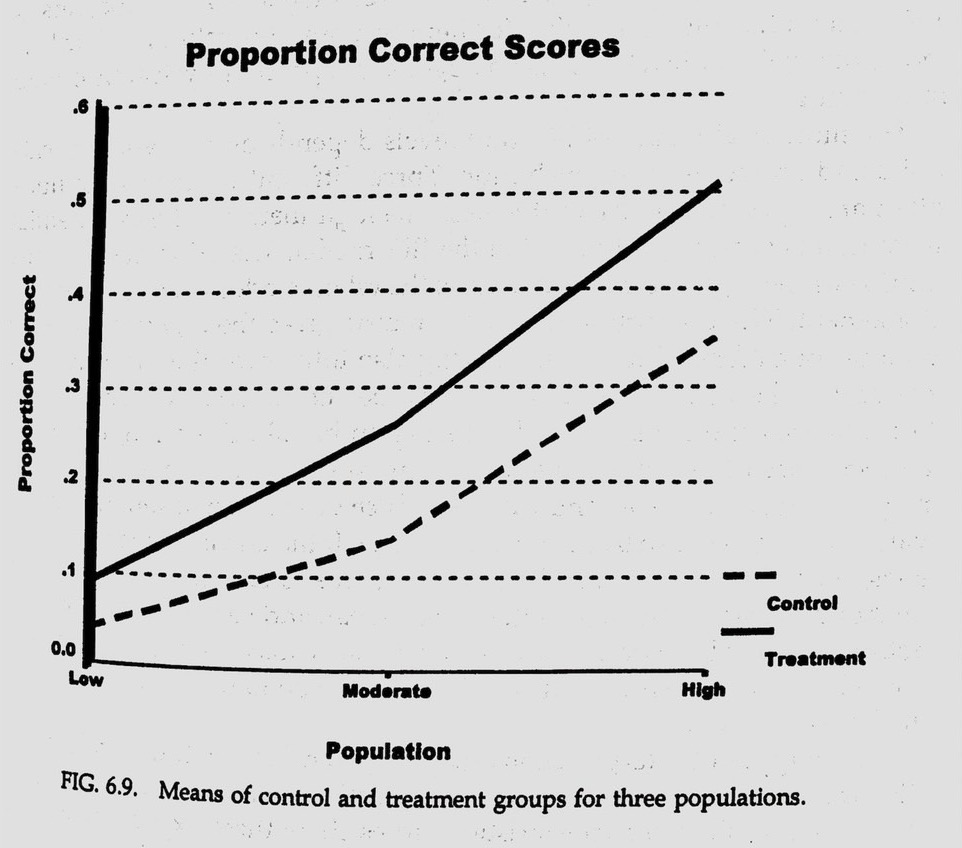]

# 13. My practice (Fig. 6.9)

trt grp m.true m.trait $$\Delta \theta$$ m.prop. $$\Delta P$$ e.prop.
cnt L -1.0 -0.987 - 0.107 - 0.077
cnt M 0.0 0.013 1.000 0.223 0.116 0.184
cnt H 1.0 1.010 0.997 0.399 0.176 0.380
trt L -0.5 -0.487 - 0.157 - 0.121
trt M 0.5 0.513 1.000 0.304 0.147 0.272
trt H 1.5 1.510 0.997 0.501 0.197 0.502

.font80[ Note. (1) set.seed(1234),
(2)m.true=mean of true. (3)m.trait=mean of trait level. (4)m.prop=mean of proportion correct. (5)e.prop= $$\rm e^{ m.trait - 1.5}/(1+e^{ m.trait - 1.5})$$ ]

## ANOVA tables

## Analysis of Variance Table
##
## Response: prp
##             Df Sum Sq Mean Sq  F value  Pr(>F)
## grp          2 30.590 15.2949 536.7894 < 2e-16 ***
## trt          1  2.731  2.7306  95.8334 < 2e-16 ***
## grp:trt      2  0.208  0.1042   3.6575 0.02599 *
## Residuals 1794 51.117  0.0285
## ---
## Signif. codes:  0 '***' 0.001 '**' 0.01 '*' 0.05 '.' 0.1 ' ' 1
## Analysis of Variance Table
##
## Response: tht
##             Df Sum Sq Mean Sq F value Pr(>F)
## grp          2 1200.0  600.00  594.86 <2e-16 ***
## trt          1  112.5  112.50  111.54 <2e-16 ***
## grp:trt      2    0.0    0.00    0.00      1
## Residuals 1794 1809.5    1.01
## ---
## Signif. codes:  0 '***' 0.001 '**' 0.01 '*' 0.05 '.' 0.1 ' ' 1

## 小疑問

• 如果改成用前述條件模擬1筆作答資料（例如 10 題的二元計分資料），卻會發現 IRT 或 CTT 都沒有交互作用？也無法很好的復原回 true score?? （不知道是模擬資料的問題或是？？）
• 模擬 6 組不同分配的資料，跑同一個 IRT 模型是否會有一些問題？？（分開跑每組的 $$\theta$$ 平均會是 0）

# Appendix

sim_dat4 <- \(sim_m, sim_t, sim_g) {
N <- 300
set.seed(1234)
tht <- rnorm(N, mean=sim_m, sd=1)
tht <- as.data.frame(tht)
tht$grp <- sim_g tht$trt <- sim_t
return(tht)
}
p_fun <- \(x){
exp(x-1.5)/(1+exp(x-1.5))
}
th_fun <- \(p){
log(p/(1-p)) + 1.5
}

prp_calc <- \(dat) {
prp <- c()
for (i in 1:nrow(dat) ) {
prp[i] <- p_fun( dat$tht[i] ) } dat$prp <- prp
return(dat)
}
# simulate data
dat_tl <- sim_dat4(sim_m=-0.5, sim_t='trt', sim_g='L')
dat_tm <- sim_dat4(sim_m=0.5, sim_t='trt', sim_g='M')
dat_th <- sim_dat4(sim_m=1.5, sim_t='trt', sim_g='H')
dat_cl <- sim_dat4(sim_m=-1, sim_t='cnt', sim_g='L')
dat_cm <- sim_dat4(sim_m=0, sim_t='cnt', sim_g='M')
dat_ch <- sim_dat4(sim_m=1, sim_t='cnt', sim_g='H')

dat <- rbind(dat_tl, dat_tm, dat_th, dat_cl, dat_cm, dat_ch)
dat$id <- seq(1:nrow(dat)) dat$id <- as.factor(dat$id) dat$grp <- as.factor(dat$grp) dat$trt <- as.factor(dat\$trt)

dat <- prp_calc(dat)

anova( lm(prp ~ grp*trt, data = dat) )
anova( lm(tht ~ grp*trt, data = dat) )